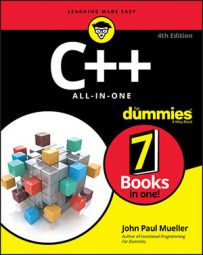##### C++ All-in-One For DummiesSaving time and effort is part of the reason you use literals. There is a shorthand way to create literals and ensure that you obtain the correct constant type. Many of the standard literals provide you with a prefix or suffix you can use to access them. Precisely how the prefix or suffix is interpreted depends on how you use it.

For example, a suffix of U could mean an unsigned int when used with an int value, while a prefix of U could mean a char32_t const pointer when used with a character string. The table shows a listing of the prefixes and suffixes that most compilers support.

Standard Prefixes and Suffixes
Data Type Prefix Suffix Resultant Type
int U or u unsigned int
int L or l long
int UL, Ul, uL, ul, LU, Lu, lU, or lu unsigned long
int LL or ll long long
int ULL, Ull, uLL, ull, LLU, LLu, llU, or llu unsigned long long
double F or f float
double L or l long double
char L wchar_t
char U char32_t
char u char16_t
String L wchar_t const*
String U char32_t const*
String u char16_t const*

Using the prefixes and suffixes can save you considerable time. The PrefixesAndSuffixes example here demonstrates how you’d employ them to create variables of various sorts.

```#include <typeinfo>
#include <cxxabi.h>
using namespace std;
using namespace abi;
char* Demangle(const char* Object)
{
int Status;
char* RealName;
RealName = __cxa_demangle(Object, 0, 0, &Status);
return RealName;
}
int main()
{
auto Int1 = 23;
auto Int2 = 23L;
auto Int3 = 23U;
auto Int4 = 23u;
auto String1 = "Hello";
auto String2 = L"Hello";
auto String3 = U"Hello";
auto String4 = u"Hello";
cout << Int1 << endl << Demangle(typeid(Int1).name()) << endl;
cout << Int2 << endl << Demangle(typeid(Int2).name()) << endl;
cout << Int3 << endl << Demangle(typeid(Int3).name()) << endl;
cout << Int4 << endl << Demangle(typeid(Int4).name()) << endl;
cout << String1 << endl << Demangle(typeid(String1).name()) << endl;
cout << String2 << endl << Demangle(typeid(String2).name()) << endl;
cout << String3 << endl << Demangle(typeid(String3).name()) << endl;
cout << String4 << endl << Demangle(typeid(String4).name()) << endl;
return 0;
}```

The Demangle() function is GCC specific. Most C++ compilers mangle (modify the spelling of) keywords and type information to make an application harder for someone to reverse-assemble (convert from machine language back into C++ source code).

In order to determine type information, you use the typeid() function to create a typeinfo structure. The name() function returns the type name found in this structure to display it onscreen. However, this name is mangled, so you must use the Demangle() function to change it back to its original readable form.

Most of the examples in this chapter rely on the auto keyword to automatically detect the variable type created by a UDL. This keyword is an important feature for newer C++ applications that make use of the new extensions that the language provides.

In this case, the code uses the auto keyword to detect the output of the literal prefix or suffix so that the variable is automatically the correct type for a situation. When you run this application, you see the following output:

```23
int
23
long
23
unsigned int
23
unsigned int
Hello
char const*
0x46e02c
wchar_t const*
0x46e038
char32_t const*
0x46e02c
char16_t const*```

Even though the data is the same in every case, the variables used to hold the data differ because of the prefix or suffix used to create the variable. Notice that the same prefix or suffix has different effects depending on the type of the variable to which it’s applied. In addition, sometimes the case of the prefix or suffix matters (as in working with a string).

Some compilers, such as GCC, include non-standard extensions for numeric data that you need to use with care. Given the popularity of GCC and the need for these number formats, you may find that you absolutely do need to use them at times. Here are some common suffix extensions provided by GCC implementations:

• d: double

• df: _Decimal32

• dd: _Decimal64

• dl: _Decimal 128

• w: __float80

• q: __float128

• i: double complex

• fi: float complex